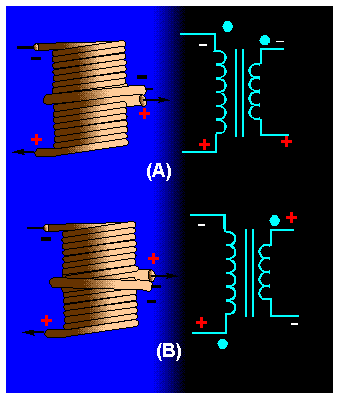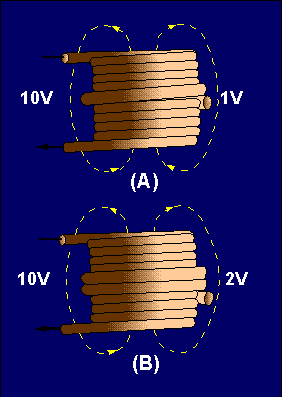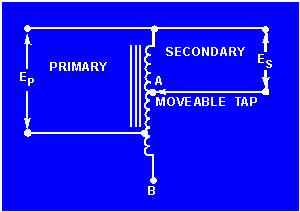SummaryCustom SearchSUMMARY As a study aid and for future reference, the important points of this chapter have been summarized below. BASIC TRANSFORMER - The basic transformer is an electrical device that transfers alternating-current energy from one circuit to another circuit by magnetic coupling of the primary and secondary windings of the transformer. This is accomplished through mutual inductance (M). The coefficient of coupling (K) of a transformer is dependent upon the size and shape of the coils, their relative positions, and the characteristic of the core between the two coils. An ideal transformer is one where all the magnetic lines of flux produced by the primary cut the entire secondary. The higher the K of the transformer, the higher is the transfer of the energy. The voltage applied to the primary winding causes current to flow in the primary. This current generates a magnetic field, generating a counter emf (cemf) which has the opposite phase to that of the applied voltage. The magnetic field generated by the current in the primary also cuts the secondary winding and induces a voltage in this winding.TRANSFORMER CONSTRUCTION - A TRANSFORMER consists of two coils of insulated wire wound on a core. The primary winding is usually wound onto a form, then wrapped with an insulating material such as paper or cloth. The secondary winding is then wound on top of the primary and both windings are wrapped with insulating material. The windings are then fitted onto the core of the transformer. Cores come in various shapes and materials. The most common materials are air, soft iron, and laminated steel. The most common types of transformers are the shell-core and the hollow-core types. The type and shape of the core is dependent on the intended use of the transformer and the voltage applied to the current in the primary winding.EXCITING CURRENT - When voltage is applied to the primary of a transformer, exciting current flows in the primary. The current causes a magnetic field to be set up around both the primary and the secondary windings. The moving flux causes a voltage to be induced into the secondary winding, countering the effects of the counter emf in the primary. PHASE - When the secondary winding is connected to a load, causing current to flow in the secondary, the magnetic field decreases momentarily. The primary then draws more current, restoring the magnetic field to almost its original magnitude. The phase of the current flowing in the secondary circuit is dependent upon the phase of the voltage impressed across the primary and the direction of the winding of the secondary. If the secondary were wound in the same direction as the primary, the phase would be the same. If wound opposite to the primary, the phase would be reversed. This is shown on a schematic drawing by the use of phasing dots. The dots mean that the leads of the primary and secondary have the same phase. The lack of phasing dots on a schematic means a phase reversal.TURNS RATIO - The TURNS RATIO of a transformer is the ratio of the number of turns of wire in the primary winding to the number of turns in the secondary winding. When the turns ratio is stated, the number representing turns on the primary is always stated first. For example, a 1:2 turns ratio means the secondary has twice the number of turns as the primary. In this example, the voltage across the secondary is two times the voltage applied to the primary.POWER AND CURRENT RATIOS - The power and current ratios of a transformer are dependent on the fact that power delivered to the secondary is always equal to the power delivered to the primary minus the losses of the transformer. This will always be true, regardless of the number of secondary windings. Using the law of power and current, it can be stated that current through the transformer is the inverse of the voltage or turns ratio, with power remaining the same or less, regardless of the number of secondaries. TRANSFORMER LOSSES - Transformer losses have two sources-copper loss and magnetic loss. Copper losses are caused by the resistance of the wire (I2R). Magnetic losses are caused by eddy currents and hysteresis in the core. Copper loss is a constant after the coil has been wound and therefore a measureable loss. Hysteresis loss is constant for a particular voltage and current. Eddy-current loss, however, is different for each frequency passed through the transformer. TRANSFORMER EFFICIENCY - The amplitude of the voltage induced in the secondary is dependent upon the efficiency of the transformer and the turns ratio. The efficiency of a transformer is related to the power losses in the windings and core of the transformer. Efficiency (in percent) equals Pout/Pin X 100. A perfect transformer would have an efficiency of 1.0 or 100%. POWER TRANSFORMER - A transformer with two or more windings wound on a laminated iron core. The transformer is used to supply stepped up and stepped down values of voltage to the various circuits in electrical equipment.AUTOTRANSFORMER - A transformer with a single winding in which the entire winding can be used as the primary and part of the winding as the secondary, or part of the winding can be used as the primary and the entire winding can be used as the secondary.AUDIO-FREQUENCY TRANSFORMER - A transformer used in audio-frequency circuits to transfer af signals from one circuit to another. RADIO-FREQUENCY TRANSFORMER - A transformer used in a radio-frequency circuit to transfer rf signals from one circuit to another. IMPEDANCE-MATCHING TRANSFORMER - A transformer used to match the impedance of the source and the impedance of the load. The mathematical relationship of the turns and impedance of the transformer is expressed by the equation:Integrated Publishing, Inc. - A (SDVOSB) Service Disabled Veteran Owned Small Business